### IMO Shortlist 2017 problem A2

Kvaliteta:
Avg: 0,0
Težina:
Avg: 6,0

Let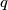$q$ be a real number. Gugu has a napkin with ten distinct real numbers written on it, and he writes the following three lines of real numbers on the blackboard: In the first line, Gugu writes down every number of the form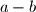$a-b$, where$a$ and$b$ are two (not necessarily distinct) numbers on his napkin. In the second line, Gugu writes down every number of the form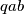$qab$, where$a$ and$b$ are two (not necessarily distinct) numbers from the first line. In the third line, Gugu writes down every number of the form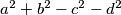$a^2+b^2-c^2-d^2$, where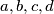$a, b, c, d$ are four (not necessarily distinct) numbers from the first line. Determine all values of$q$ such that, regardless of the numbers on Gugu's napkin, every number in the second line is also a number in the third line.

Izvor: https://www.imo-official.org/problems/IMO2017SL.pdf